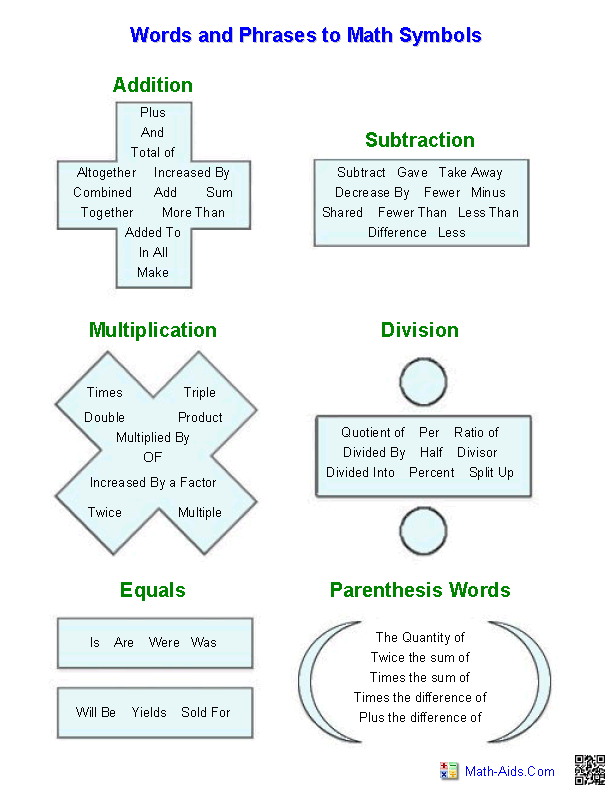#Pre-Algebra Worksheets

## Algebraic Expressions Worksheets

Here is a graphic preview for all of the Algebraic Expressions Worksheets. You can select different variables to customize these Algebraic Expressions Worksheets for your needs. The Algebraic Expressions Worksheets are randomly created and will never repeat so you have an endless supply of quality Algebraic Expressions Worksheets to use in the classroom or at home.

Our Algebraic Expressions Worksheets are free to download, easy to use, and very flexible.

These Algebraic Expressions Worksheets are a good resource for students in the 5th Grade through the 8th Grade.

Click here for a Detailed Description of all the Algebraic Expressions Worksheets.

## Quick Link for All Algebraic Expressions Worksheets

Click the image to be taken to that Algebraic Expressions Worksheets.

##### Words to AlgebraicExpression Handout##### Algebraic ExpressionsTranslate Phrases Worksheets##### Algebraic ExpressionsSimplifying Variables##### Algebraic ExpressionsThe Distributive Property##### Algebraic ExpressionsEvaluating One Variable##### Algebraic ExpressionsEvaluating Two Variables## Detailed Description for All Algebraic Expressions Worksheets

Words to Algebraic Expressions
These Algebraic Expressions Worksheets will produce a great handout to help students learn the symbols for different words and phrases in word problems. These Algebraic Expressions Worksheets are a good resource for students in the 5th Grade through the 8th Grade.

Translate Phrases Worksheets
These Algebraic Expressions Worksheets will create word problems for the students to translate into an algebraic statement. These Algebraic Expressions Worksheets are a good resource for students in the 5th Grade through the 8th Grade.

Simplifying Variables Expressions Worksheets
These Algebraic Expressions Worksheets will create algebraic statements for the student to simplify. You may select from 2, 3, or 4 terms with addition, subtraction, and multiplication. These Algebraic Expressions Worksheets are a good resource for students in the 5th Grade through the 8th Grade.

The Distributive Property Worksheets
These Algebraic Expressions Worksheets will create algebraic statements for the student to simplify. You may select from 3 and 4 terms with addition, subtraction, and multiplication. These Algebraic Expressions Worksheets are a good resource for students in the 5th Grade through the 8th Grade.

Evaluating One Variable Expressions Worksheets
These Algebraic Expressions Worksheets will create algebraic statements with one variable for the student to evaluate. You may select from 2, 3 and 4 terms with addition, subtraction, multiplication, and division. These Algebraic Expressions Worksheets are a good resource for students in the 5th Grade through the 8th Grade.

Evaluating Two Variables Expressions Worksheets
These Algebraic Expressions Worksheets will create algebraic statements with two variables for the student to evaluate. You may select from 2, 3 and 4 terms with addition, subtraction, multiplication, and division. These Algebraic Expressions Worksheets are a good resource for students in the 5th Grade through the 8th Grade.